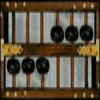# Resources tagged with: Calculators

Filter by: Content type:
Age range:
Challenge level:

### There are 19 results

Broad Topics > Information and Communications Technology > Calculators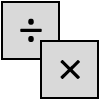### Four Go

##### Age 7 to 11 Challenge Level:

This challenge is a game for two players. Choose two of the numbers to multiply or divide, then mark your answer on the number line. Can you get four in a row?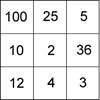### Four Go for Two

##### Age 7 to 11 Challenge Level:

Four Go game for an adult and child. Will you be the first to have four numbers in a row on the number line?### One Million to Seven

##### Age 7 to 11 Challenge Level:

Start by putting one million (1 000 000) into the display of your calculator. Can you reduce this to 7 using just the 7 key and add, subtract, multiply, divide and equals as many times as you like?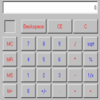### Calculating the Difference: A Discussion of the Use of Calculators in the English Primary Classroom.

##### Age 5 to 11

Clare Green looks at the role of the calculator in the teaching and learning of primary mathematics.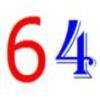##### Age 7 to 11 Challenge Level:

Write the numbers up to 64 in an interesting way so that the shape they make at the end is interesting, different, more exciting ... than just a square.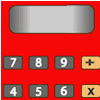### Forgot the Numbers

##### Age 7 to 11 Challenge Level:

On my calculator I divided one whole number by another whole number and got the answer 3.125. If the numbers are both under 50, what are they?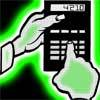### Reverse Trick

##### Age 7 to 11 Challenge Level:

Tell your friends that you have a strange calculator that turns numbers backwards. What secret number do you have to enter to make 141 414 turn around?### A Conversation Piece

##### Age 7 to 11 Challenge Level:

Take the number 6 469 693 230 and divide it by the first ten prime numbers and you'll find the most beautiful, most magic of all numbers. What is it?### Book Codes

##### Age 7 to 11 Challenge Level:

Look on the back of any modern book and you will find an ISBN code. Take this code and calculate this sum in the way shown. Can you see what the answers always have in common?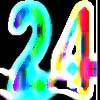### Special 24

##### Age 7 to 11 Challenge Level:

Find another number that is one short of a square number and when you double it and add 1, the result is also a square number.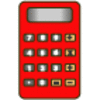### Clever Keys

##### Age 7 to 11 Short Challenge Level:

On a calculator, make 15 by using only the 2 key and any of the four operations keys. How many ways can you find to do it?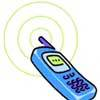### Mobile Numbers

##### Age 5 to 11 Challenge Level:

In this investigation, you are challenged to make mobile phone numbers which are easy to remember. What happens if you make a sequence adding 2 each time?### Exploring Wild & Wonderful Number Patterns

##### Age 7 to 11 Challenge Level:

EWWNP means Exploring Wild and Wonderful Number Patterns Created by Yourself! Investigate what happens if we create number patterns using some simple rules.##### Age 11 to 14 Challenge Level:

Make a set of numbers that use all the digits from 1 to 9, once and once only. Add them up. The result is divisible by 9. Add each of the digits in the new number. What is their sum? Now try some. . . .### Largest Product

##### Age 11 to 14 Challenge Level:

Which set of numbers that add to 10 have the largest product?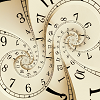### Decimal Time

##### Age 11 to 14 Challenge Level:

Use the clocks to investigate French decimal time in this problem. Can you see how this time system worked?### Round and Round and Round

##### Age 11 to 14 Challenge Level:

Where will the point stop after it has turned through 30 000 degrees? I took out my calculator and typed 30 000 ÷ 360. How did this help?